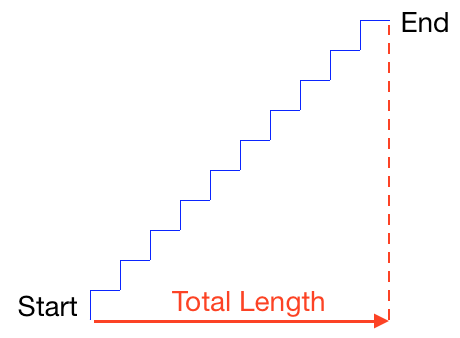Select Page

## Staircase

### Drawing a Staircase

Below is a script that draws a staircase.Which block determines how long each stair is?

Which block determines how many stairs there are?

What is the total length of the staircase (shown by the red arrow), in steps? (Note: In this case, “steps” refers to the units of distance in Scratch, not the number of unique stairs created in the drawing.)

You have used 0 of 5 attemptsSome problems have options such as save, reset, hints, or show answer. These options follow the Submit button.

### Scaling Staircases

What numbers should go in the blanks in these scripts so that they make staircases with the same length as the staircase above?

## Script A## Script BHow many stairs are there in the staircase drawn by Script A?

How many stairs are there in the staircase drawn by Script B?

How many units wide are the stairs in the staircase drawn by Script A?

How many units wide are the stairs in the staircase drawn by Script B?

You have used 0 of 5 attemptsSome problems have options such as save, reset, hints, or show answer. These options follow the Submit button.

### Matching Perimeters

Fill in the blanks in Scripts B, C, and D below so that they draw shapes with the same perimeter as Script A and only turn 360 degrees total. Remember, perimeter is the number of steps on each side of a shape, so Script A has a perimeter of 320 steps.

## Script A## Script BWhat number goes in?

Script CWhat number goes in?

What number goes in?

Script DWhat number goes in??